Fundamental OperationsNext: Formal Definition of Up: The Relational Algebra Previous: The Relational Algebra

Fundamental Operations

1. The Select Operation

Select selects tuples that satisfy a given predicate. Select is denoted by a lowercase Greek sigma (), with the predicate appearing as a subscript. The argument relation is given in parentheses following the.

For example, to select tuples (rows) of the borrow relation where the branch is ``SFU'', we would write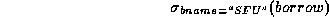Let Figure 3.3 be the borrow and branch relations in the banking example.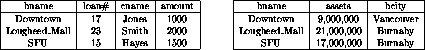Figure 3.3:   The borrow and branch relations.

The new relation created as the result of this operation consists of one tuple: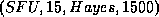.

We allow comparisons using =,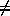, <,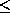, > and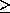in the selection predicate.

We also allow the logical connectives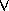(or) and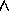(and). For example: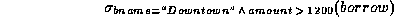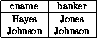Figure 3.4:   The client relation.

Suppose there is one more relation, client, shown in Figure 3.4, with the scheme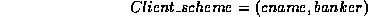we might write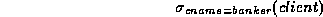to find clients who have the same name as their banker.

2. The Project Operation

Project copies its argument relation for the specified attributes only. Since a relation is a set, duplicate rows are eliminated.

Projection is denoted by the Greek capital letter pi (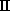). The attributes to be copied appear as subscripts.

For example, to obtain a relation showing customers and branches, but ignoring amount and loan#, we write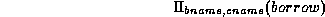We can perform these operations on the relations resulting from other operations.

To get the names of customers having the same name as their bankers,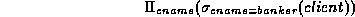Think of select as taking rows of a relation, and project as taking columns of a relation.

3. The Cartesian Product Operation

The cartesian product of two relations is denoted by a cross (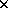), written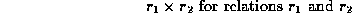The result of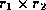is a new relation with a tuple for each possible pairing of tuples from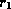and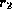.

In order to avoid ambiguity, the attribute names have attached to them the name of the relation from which they came. If no ambiguity will result, we drop the relation name.

The result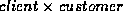is a very large relation. Ifhas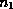tuples, andhas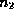tuples, then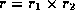will have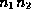tuples.

The resulting scheme is the concatenation of the schemes ofand, with relation names added as mentioned.

To find the clients of banker Johnson and the city in which they live, we need information in both client and customer relations. We can get this by writing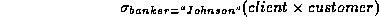However, the customer.cname column contains customers of bankers other than Johnson. (Why?)

We want rows where client.cname = customer.cname. So we can write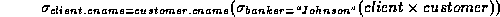to get just these tuples.

Finally, to get just the customer's name and city, we need a projection: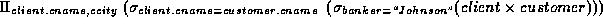4. The Rename Operation

The rename operation solves the problems that occurs with naming when performing the cartesian product of a relation with itself.

Suppose we want to find the names of all the customers who live on the same street and in the same city as Smith.

We can get the street and city of Smith by writing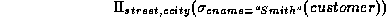To find other customers with the same information, we need to reference the customer relation again: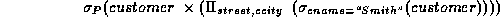where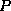is a selection predicate requiring street and ccity values to be equal.

Problem: how do we distinguish between the two street values appearing in the Cartesian product, as both come from a customer relation?
Solution: use the rename operator, denoted by the Greek letter rho (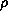).

We write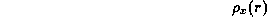to get the relationunder the name of.

If we use this to rename one of the two customer relations we are using, the ambiguities will disappear.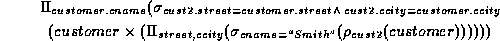5. The Union Operation

The union operation is denoted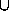as in set theory. It returns the union (set union) of two compatible relations.

For a union operation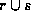to be legal, we require that

•andmust have the same number of attributes.
• The domains of the corresponding attributes must be the same.

To find all customers of the SFU branch, we must find everyone who has a loan or an account or both at the branch.

We need both borrow and deposit relations for this: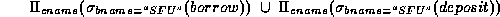As in all set operations, duplicates are eliminated, giving the relation of Figure 3.5(a).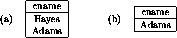Figure 3.5:   The union and set-difference operations.

6. The Set Difference Operation

Set difference is denoted by the minus sign (). It finds tuples that are in one relation, but not in another.

Thusresults in a relation containing tuples that are inbut not in.

To find customers of the SFU branch who have an account there but no loan, we write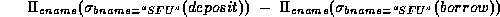The result is shown in Figure 3.5(b).

We can do more with this operation. Suppose we want to find the largest account balance in the bank.
Strategy:

• Find a relationcontaining the balances not the largest.
• Compute the set difference ofand the deposit relation.

To find, we write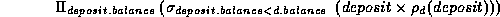This resulting relation contains all balances except the largest one. (See Figure 3.6(a)).

Now we can finish our query by taking the set difference: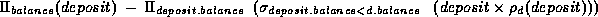Figure 3.6(b) shows the result.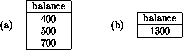Figure 3.6:   Find the largest account balance in the bank.Next: Formal Definition of Up: The Relational Algebra Previous: The Relational Algebra

Page created and maintained by Osmar R. Zaï ane
Last Update: Wed Sep 20 15:45:57 PDT 1995